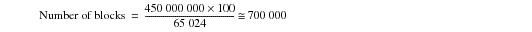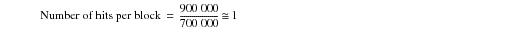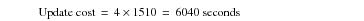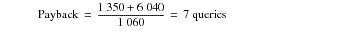# Example of Computing a Payback Factor for an Aggregate Join Index - Teradata Database

Product
Release Number
15.10
Language
English (United States)
Last Update
2018-10-06
Product Category
Software

### Example of Computing a Payback Factor for an Aggregate Join Index

In the scenario supporting this example, a 30 day period is used for the analysis. You do not regularly create and drop join indexes in your production environment, but you want to calculate the cost of creating the index.

The query to be supported by the join index joins two tables and uses aggregation. The join is made on the primary index of both tables. This is the definition of an in-place aggregate join index.

The 2‑node production system used to generate these numbers had the following general configuration.

• Two 550 MHz 4-processor nodes with 1 MB cache and 2 GB memory per node
• RAID1 (mirrored) disk arrays with a segment size of 968
• BYNET 2
• 20 AMPs, each with 2 LUNs (4 physical disks)
• 4 network-attached PEs
• The following table indicates the demographics of your data.

 Data Characteristic Table A Table B Row length (bytes) 100 Cardinality 450 million rows 600 million rows Number of rows for each value in the aggregating column 10,000 Number of rows updated weekly 900,000 Not applicable Data block size 64 KB

The join indexes were created on base tables, A and B with the following characteristics.

• Row size of 100 bytes (except where row size is manipulated as a variable)
• Initial block size of 65,024 bytes
• Base and join index table cardinalities
•
 Type of Join          Index Cardinality of Join Index            Table (Rows) Cardinality of Base Table            100A (Rows) Cardinality of Base Table            100B (Rows) Simple 45 x 106 45 x 106 60 x 106 Aggregate 45 x 102

The following table lists the tunable parameter settings used on the test system throughout the test cycle.

 Parameter Setting Comment ReadAhead flag setting TRUE Disk I/O DBS Don't Cache flag setting TRUE Use DBC Cache Threshold setting DBC Cache Threshold 10 Default setting FSG Cache Percent 80 Default setting RSS logging settings SPMA IPMA SVPR 15 second logging interval Datablock size 64 KB Free Space Percent 0 Mini Cyl Pack 10 cylinders Low Cylinder

How many times must the query be performed to recover the cost in the first month? Here is the procedure.

1 Estimate the benefit.

The benefit should be approximately one order of magnitude greater than the figure generated by this study because there are that many more rows in the base tables, while all other factors are identical.

Examine the table in the topic “Creation and Elapsed Query Times for Different Join Indexes” on page 474. The measured benefit is 106 seconds. Multiply this by 10 to produce the estimated benefit for your configuration, which is 1060 seconds.

2 Estimate the cost of creation.

Examine the table in the topic “Creation and Elapsed Query Times for Different Join Indexes” on page 474. The cost of creation for an in-place aggregate join index is seen to be 135 seconds. Scale this value up by an order of magnitude for the same reason as Step 1.

The estimated cost of creation is 1350 seconds.

3 Estimate the approximate number of hits per block.

You update 900,000,100-byte rows in a 450 million row table weekly.

Calculate the number of blocks these 450 million 100-byte rows occupy. The equation is as follows.Determine the approximate hit rate. With approximately 700,000 blocks and 900,000 rows to be updated weekly, the hit rate is determined as follows.4 Determine the update cost.

In a 30 day period, the table will be updated four times.

Suppose that the cost of this update is 151 seconds for the test system.

Scale this number up by an order of magnitude and multiply it by four weeks to obtain the approximate update cost.5 Calculate the payback factor.If the query for which this join index was designed is run twice weekly, it recovers the time spent creating and maintaining it.